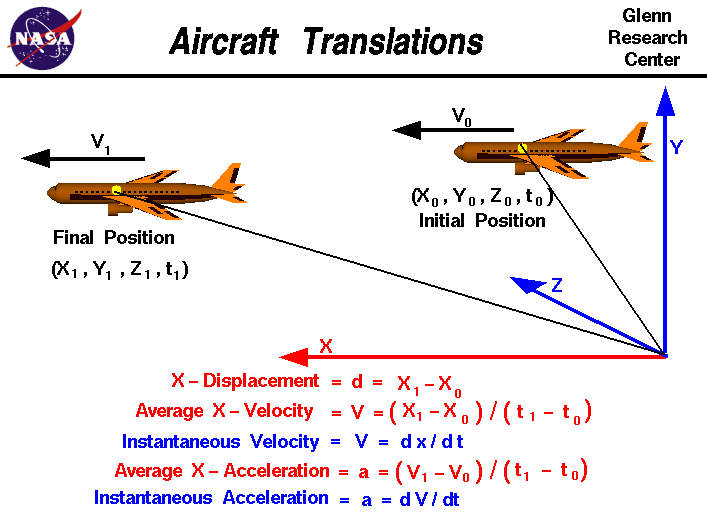+ Text Only Site
+ Non-Flash Version
+ Contact GlennWe live in world that is defined by three spatial dimensions and one time dimension. Objects can move within this domain in two ways. An object can translate, or change location, from one point to another. And an object can rotate, or change its attitude. In general, the motion of an object involves both translation and rotation. The motion of an aircraft is particularly complex because the rotations and translations are coupled together; a rotation affects the magnitude and direction of the forces which affect translations. On this page we will consider only the translation of an aircraft within our domain. We can specify the location of our aircraft at any time t by specifying three coordinates x, y, and z on an orthogonal coordinate system. An orthogonal coordinate system has each of its coordinate directions perpendicular to all other coordinate directions. Initially, our aircraft is at point "0", with coordinates x0, y0, and z0 at time t0. In general, the aircraft moves through the domain until at some later time t1 the aircraft is at point "1" with coordinates x1, y1, and z1. We can specify the displacement - d in each coordinate direction by the difference in coordinate from point "0" to point "1". The x-displacement equals (x1 - x0), the y-displacement equals (y1 - y0), and the z-displacement equals (z1 - z0). For simplicity on the slide we are only going to consider the x coordinate. d = x1 - x0 The total displacement is a vector quantity, which means that the displacement has a size and a direction associated with it. The direction is from point "0" to point "1". The individual x-, y-, and z-displacements are the components of the displacement vector in the coordinate directions. All of the quantities derived from the displacement are also vector quantities. The velocity -V of the aircraft through the domain is the change of the location with respect to time. In the X - direction, the average velocity is the displacement divided by the time interval: V = (x1 - x0) / (t1 - t0) This is an average velocity and the aircraft could speed up and slow down between points "0" and "1". At any instant, the aircraft could have a velocity that is different than the average. If we shrink the time difference down to a very small (differential) size, we can define the instantaneous velocity to be the differential change in position divided by the differential change in time; V = dx / dt where the symbol d / dt is the differential from calculus. So when we initially specified the location of our aircraft with x0, y0, z0, and t0 coordinates, we could also specify an initial instantaneous velocity V0. Likewise at the final position x1, y1, z1, and t1, the velocity changes to a velocity V1. Again, for simplicity, we are considering only the x-component of the velocity. In reality, the aircraft velocity changes in all three directions. Velocity is a vector quantity and has both a magnitude and a direction. The acceleration (a) of the aircraft through the domain is the change of the velocity with respect to time. In the X - direction, the average acceleration is the change in velocity divided by the time interval: a = (V1 - V0) / (t1 - t0) As with the velocity, this is an average acceleration. At any instant, the aircraft could have an acceleration that is different than the average. If we shrink the time difference down to a very small (differential) size, we can define the instantaneous acceleration to be the differential change in velocity divided by the differential change in time: a = dv / dt From Newton's second law of motion, we know that forces on an object produce accelerations. If we can determine the forces on an aircraft, and how the forces change with time, we can use the equations presented on this slide to determine the acceleration, velocity, and location of the aircraft as a function of time. Activities:Guided ToursForces, Torques and Motion:Basic Aircraft Motion:Cruising Aircraft:Navigation ..Beginner's Guide Home Page+ Inspector General Hotline + Equal Employment Opportunity Data Posted Pursuant to the No Fear Act + Budgets, Strategic Plans and Accountability Reports + Freedom of Information Act + The President's Management Agenda + NASA Privacy Statement, Disclaimer, and Accessibility CertificationEditor: Nancy Hall NASA Official: Nancy Hall Last Updated: May 13 2021 + Contact Glenn